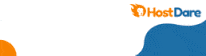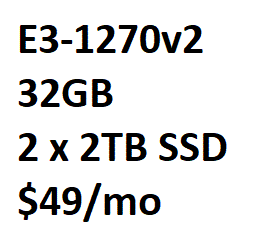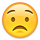#### Howdy, Stranger!

It looks like you're new here. If you want to get involved, click one of these buttons!# Test the disk I/O of your VPS

BuyVM OpenVZ (256/512 MB RAM, 30 GB HDD, 1 TB B/W)

```dd if=/dev/zero of=test bs=64k count=16k conv=fdatasync
16384+0 records in
16384+0 records out
1073741824 bytes (1.1 GB) copied, 5.78672 s, 186 MB/s```
```dd if=/dev/zero of=test bs=64k count=16k conv=fdatasync
16384+0 records in
16384+0 records out
1073741824 bytes (1.1 GB) copied, 5.93356 s, 181 MB/s```

Hostigation KVM (128 MB RAM, 10 GB HDD, 500 GB B/W)

```dd if=/dev/zero of=test bs=64k count=16k conv=fdatasync
16384+0 records in
16384+0 records out
1073741824 bytes (1.1 GB) copied, 6.29459 s, 171 MB/s```
```dd if=/dev/zero of=test bs=64k count=16k conv=fdatasync
16384+0 records in
16384+0 records out
1073741824 bytes (1.1 GB) copied, 11.1185 s, 96.6 MB/s```

eNetSouth OpenVZ (786/1024 MB RAM, 20 GB HDD, 1000 GB B/W)

```dd if=/dev/zero of=test bs=64k count=16k conv=fdatasync
16384+0 records in
16384+0 records out
1073741824 bytes (1.1 GB) copied, 31.4363 s, 34.2 MB/s```
```dd if=/dev/zero of=test bs=64k count=16k conv=fdatasync
16384+0 records in
16384+0 records out
1073741824 bytes (1.1 GB) copied, 13.1464 s, 81.7 MB/s```
```dd if=/dev/zero of=test bs=64k count=16k conv=fdatasync
16384+0 records in
16384+0 records out
1073741824 bytes (1.1 GB) copied, 13.4436 s, 79.9 MB/s```

WhyNOTaVPS OpenVZ (786/1024 MB RAM, 33 GB HDD, 444 GB B/W)

```dd if=/dev/zero of=test bs=64k count=16k conv=fdatasync
16384+0 records in
16384+0 records out
1073741824 bytes (1.1 GB) copied, 24.3903 s, 44.0 MB/s```

• My MyCpanel Free VPS:

[root@l90 ~]# dd if=/dev/zero of=test bs=64k count=16k conv=fdatasync
16384+0 records in
16384+0 records out
1073741824 bytes (1.1 GB) copied, 20.6291 seconds, 52.0 MB/s

• KiloServe 1GB RAM Low End Box special

```dd if=/dev/zero of=/tmp/output.img bs=64k count=16k conv=fdatasync
16384+0 records in
16384+0 records out
1073741824 bytes (1.1 GB) copied, 4.27653 seconds, 251 MB/s
```

RapidoVPS 256MB RAM \$2.50 month special

```dd if=/dev/zero of=test bs=64k count=16k conv=fdatasync
16384+0 records in
16384+0 records out
1073741824 bytes (1.1 GB) copied, 33.215 s, 32.1 MB/s
```

I would post my W2Servers benchmark ... if the stupid VPS was running but it's been down so long I don't even bother anymore.

• not too fast :
dd if=/dev/zero of=test bs=64k count=16k conv=fdatasync

```16384+0 records in
16384+0 records out
1073741824 bytes (1.1 GB) copied, 18.7822 seconds, 57.2 MB/s
```
• edited August 2011
```dd if=/dev/zero of=test bs=64k count=16k conv=fdatasync
16384+0 records in
16384+0 records out
1073741824 bytes (1.1 GB) copied, 18.2208 seconds, 58.9 MB/s
```

``````dd if=/dev/zero of=test bs=64k count=16k conv=fdatasync
16384+0 records in
16384+0 records out
1073741824 bytes (1.1 GB) copied, 6.10176 seconds, 176 MB/s
``````
• hostigation 64 MB

```dd if=/dev/zero of=test bs=64k count=16k conv=fdatasync
```
```16384+0 records in
16384+0 records out
1073741824 bytes (1.1 GB) copied, 9.86716 s, 109 MB/s
```
Thanked by 1blazerunner44
• TurnKey Internet

```dd if=/dev/zero of=test bs=64k count=16k conv=fdatasync
16384+0 records in
16384+0 records out
1073741824 bytes (1.1 GB) copied, 64.3875 s, 16.7 MB/s
```

Hetzner

```dd if=/dev/zero of=test bs=64k count=16k conv=fdatasync
16384+0 records in
16384+0 records out
1073741824 bytes (1.1 GB) copied, 112.157 s, 9.6 MB/s
```

...not so lowendbox but very shity..

• @asf, which plan do you have with turnkey? (that's very very slow disk I/O)

• @tommy
Some prehistoric wht custom plan• A little over a week later, the same BuyVM OpenVZ-128MB:

``````dd if=/dev/zero of=test bs=64k count=16k conv=fdatasync
16384+0 records in
16384+0 records out
1073741824 bytes (1.1 GB) copied, 43.9629 seconds, 24.4 MB/s
``````

Ouch.

• Hi Marcel,

Could you log a ticket? It's quite possible there's a bad drive, but we'll need to track it firstThanks,

Francisco

• Chicago VPS 1GB Ram

```dd if=/dev/zero of=test bs=64k count=16k conv=fdatasync
16384+0 records in
16384+0 records out
1073741824 bytes (1.1 GB) copied, 15.1019 s, 71.1 MB/s```
• A fresh Rackspace VPS lol

```# dd if=/dev/zero of=test bs=64k conv=fdatasync count=20k
20480+0 records in
20480+0 records out
1342177280 bytes (1.3 GB) copied, 4.39452 s, 305 MB/s
```

My buyvm is starting to degrade xD but still the fastest of all my LEBs

```dd if=/dev/zero of=test bs=64k conv=fdatasync count=10k
10240+0 records in
10240+0 records out
671088640 bytes (671 MB) copied, 7.91368 s, 84.8 MB/s
```
• Francisco said: Could you log a ticket? It's quite possible there's a bad drive, but we'll need to track it firstWill do. Please don't suspend my account and delete all the backups ;D

• Criperz Prodigy 128meg annual:

```~# dd if=/dev/zero of=test bs=64k count=16k conv=fdatasync
16384+0 records in
16384+0 records out
1073741824 bytes (1.1 GB) copied, 114.226 s, 9.4 MB/s
```

Oversold much?! Very variable, same test reported 1.9 MB/s a minute later.

```:~# dd if=/dev/zero of=test bs=64k count=16k conv=fdatasync
16384+0 records in
16384+0 records out
1073741824 bytes (1.1 GB) copied, 7.92439 s, 135 MB/s
```

```~# dd if=/dev/zero of=test bs=64k count=16k conv=fdatasync
16384+0 records in
16384+0 records out
1073741824 bytes (1.1 GB) copied, 7.06734 s, 152 MB/s
```

QualityServers 128meg annual (from the recent deal):

```~# dd if=/dev/zero of=test bs=64k count=16k conv=fdatasync
16384+0 records in
16384+0 records out
1073741824 bytes (1.1 GB) copied, 39.2065 s, 27.4 MB/s
```

bHosts 1.5gb:

```dd if=/dev/zero of=test bs=64k count=16k conv=fdatasync
16384+0 records in
16384+0 records out
1073741824 bytes (1.1 GB) copied, 36.7724 s, 29.2 MB/s
```
• evorack 128 xen (not as impressive as other but very stable, no complain):

``````dd if=/dev/zero of=test bs=64k conv=fdatasync count=20k
20480+0 records in
2 0480+0 records out
1342177280 bytes (1.3 GB) copied, 24.7226 s, 54.3 MB/s
``````

vooserver 128 xen (just like evorack, no complain):

``````dd if=/dev/zero of=test bs=64k conv=fdatasync count=20k
20480+0 records in
20480+0 records out
1342177280 bytes (1.3 GB) copied, 22.2575 s, 60.3 MB/s
``````

edis 128 kvm (always have great io, I wish they have better billing/support panel):

``````dd if=/dev/zero of=test bs=64k conv=fdatasync count=6k
6144+0 records in
6144+0 records out
402653184 bytes (403 MB) copied, 2.20145 s, 183 MB/s
``````

bitcable 256 kvm (new, for test):

``````dd if=/dev/zero of=test bs=64k conv=fdatasync count=20k
20480+0 records in
20480+0 records out
1342177280 bytes (1.3 GB) copied, 11.8234 s, 114 MB/s
``````

securedragon 96 xen:

``````dd if=/dev/zero of=test bs=64k conv=fdatasync count=20k
20480+0 records in
20480+0 records out
1342177280 bytes (1.3 GB) copied, 24.0986 s, 55.7 MB/s
``````

``````dd if=/dev/zero of=test bs=64k count=16k conv=fdatasync
16384+0 records in
16384+0 records out
1073741824 bytes (1.1 GB) copied, 6.555 s, 164 MB/s
``````
• CripperZ 64 MB

```dd if=/dev/zero of=test bs=64k count=16k conv=fdatasync
16384+0 records in
16384+0 records out
1073741824 bytes (1.1 GB) copied, 71.2935 s, 15.1 MB/s```

CripperZ 128 MB

```dd if=/dev/zero of=test bs=64k count=16k conv=fdatasync
16384+0 records in
16384+0 records out
1073741824 bytes (1.1 GB) copied, 73.2753 s, 14.7 MB/s```

123Systems 192 MB

```dd if=/dev/zero of=test bs=64k count=16k conv=fdatasync
16384+0 records in
16384+0 records out
1073741824 bytes (1.1 GB) copied, 25.0444 s, 42.9 MB/s```

123Systems 512 MB

```dd if=/dev/zero of=test bs=64k count=16k conv=fdatasync
16384+0 records in
16384+0 records out
1073741824 bytes (1.1 GB) copied, 36.6829 seconds, 29.3 MB/s```

SpotVPS 1 GB

```dd if=/dev/zero of=test bs=64k count=16k conv=fdatasync
16384+0 records in
16384+0 records out
1073741824 bytes (1.1 GB) copied, 24.0437 seconds, 44.7 MB/s```

Up2VPS 1 GB

```dd if=/dev/zero of=test bs=64k count=16k conv=fdatasync
16384+0 records in
16384+0 records out
1073741824 bytes (1.1 GB) copied, 17.3729 s, 61.8 MB/s```

IPAPco SuperVPS 4 GB

``` dd if=/dev/zero of=test bs=64k count=16k conv=fdatasync
16384+0 records in
16384+0 records out
1073741824 bytes (1.1 GB) copied, 14.8628 s, 72.2 MB/s```

IPAPco SuperVPS 2GB

```dd if=/dev/zero of=test bs=64k count=16k conv=fdatasync
16384+0 records in
16384+0 records out
1073741824 bytes (1.1 GB) copied, 26.5291 s, 40.5 MB/s```

Cripperz i/o is very disappointing. Also funny that my cheaper 123Systems one is faster than the more expensive one.

• damn buyvm 128 mb, which is the cheapest out of all of them, is smoking everyone else lol

my question is (out of curiosity): what affects disk i/o speed on a vps?

• Other clients in the node.

Database trashing

Overselling -> Swapping

Hardware configuration (RAID or not, and what disks)

• I'm still wondering why we should use bs=64k for dd test? is this value some kind of standard? if it is, why 64k?

• SpotVPS - 256MB

``````dd if=/dev/zero of=test bs=64k count=16k conv=fdatasync
16384+0 records in
16384+0 records out
1073741824 bytes (1.1 GB) copied, 25.6696 s, 41.8 MB/s
``````

``````dd if=/dev/zero of=test bs=64k count=16k conv=fdatasync
16384+0 records in
16384+0 records out
1073741824 bytes (1.1 GB) copied, 11.1968 s, 95.9 MB/s
``````

FeHosting - 256MB OpenVZ

``````dd if=/dev/zero of=test bs=64k count=16k conv=fdatasync
16384+0 records in
16384+0 records out
1073741824 bytes (1.1 GB) copied, 27.7938 s, 38.6 MB/s
``````
• @circus - it's a reasonable middle grounds for most RAID stripe sizes and if the disk is SSD or mechanical. SSD's can handle say, a 4k block size, where as a mechanical drive won't be able to handle that kind of IOP's without giving really poor results (like 2 - 3MB/sec per disk kinda crap)

Francisco

Thanked by 1circus
• 123Systems - 192MB OpenVZ (Dallas)

```dd if=/dev/zero of=test bs=64k count=16k conv=fdatasync
16384+0 records in
16384+0 records out
1073741824 bytes (1.1 GB) copied, 20.8858 s, 51.4 MB/s
```

123Systems - 256MB OpenVZ(Chicago)

```dd if=/dev/zero of=test bs=64k count=16k conv=fdatasync
16384+0 records in
16384+0 records out
1073741824 bytes (1.1 GB) copied, 41.675 s, 25.8 MB/s
```

```dd if=/dev/zero of=test bs=64k count=16k conv=fdatasync
16384+0 records in
16384+0 records out
1073741824 bytes (1.1 GB) copied, 6.13831 s, 175 MB/s
```
• 1073741824 bytes (1.1 GB) copied, 17.421 seconds, 61.6 MB/s

Fuck this is one of my dedicated servers running in Raid 1 if I remember, it's not very loaded, how can I "fix" this?

• How many disks are in the array? just 2?

Francisco

• ztec said: Fuck this is one of my dedicated servers running in Raid 1 if I remember

io wise, raid 1 is no different than no raid at all CMIIW and 61 MB/s is normal for sata ^_^ drive

my desktop:

``````dd if=/dev/zero of=test bs=64k count=16k conv=fdatasync
16384+0 records in
16384+0 records out
1073741824 bytes (1.1 GB) copied, 143.523 s, 7.5 MB/s
``````

something must be really really wrongedit: It was only a bad cable, thank god.

``````dd if=/dev/zero of=test bs=64k count=16k conv=fdatasync
16384+0 records in
16384+0 records out
1073741824 bytes (1.1 GB) copied, 13.195 s, 81.4 MB/s
``````
• ztec said: 1073741824 bytes (1.1 GB) copied, 17.421 seconds, 61.6 MB/s

That's pretty normal for your typical RAID1 SATA drive dedicated server.

This is why you'll often see VPS hitting 150 and even 250 MB/s disk writes... it's due to the multiple disk spindles. If you look at the tests above, most of us VPS companies use up to 16 hard drives in our RAID arrays compared to your dedicated running 2 drives in RAID1 (mirror).

And nobody even posts read speeds but it's not uncommon to get 1GB/s+ reads on a quality VPS.

A dedicated isn't necessarily faster than a VPS.

Dollar for dollar, every one of our VPS' would whup a dedicated in processing power and disk I/O• @kiloserve: Samsung's SpinPoint F3, WD's Black Caviar and RE4 all write/read above 100 MB/s. A dedicated isn't necessarily faster than a VPS, but you can atleast use all resources, not only burst them.

Thanked by 1Brandon_eNetSouth
• You won't commonly find the Spinpoints and WD Blacks in dedicated servers.

Most dedicateds use the WD RE3 drives or standard desktop drives which were about 60-70 Mb/s.

Some providers now are using the RE4's.

I'm guessing ztec has an RE3 or older desktop drive and that is about the right speed for those drives.

Thanked by 1Brandon_eNetSouth
This discussion has been closed.